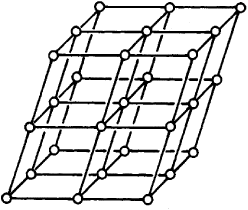# Bravais Lattice

Also found in: Dictionary, Thesaurus, Wikipedia.

## Bravais lattice

[brə′vā ′lad·əs]
(crystallography)
One of the 14 possible arrangements of lattice points in space such that the arrangement of points about any chosen point is identical with that about any other point.

## Bravais Lattice

a type of spatial crystal lattice first described by the French scientist A. Bravais in 1848. Bravais expressed the hypothesis that spatial crystal lattices are constructed of regularly spaced node-points (where the atoms are located) that can be obtained by repeating a given point by means of parallel transpositions (translations). (See Figure 1.) When straight lines and planes are constructedFigure 1. Structural diagram of a spatial crystal lattice drawn by means of parallel translations

through these points, the spatial lattice becomes divided into equal parallelepipeds (cells). There are a total of 14 types of such lattices, by which the structure of any crystal can be described in the first approximation. Bravais lattices are divided into four types (Figure 2):

(1) primitive, in which the points occur only at the vertices of the parallelepiped;

(2) base-centered, having an additional point in each of the centers of two opposite faces;

(3) volume-centered, in which a point in the center of the cell is added to the primitive type;

(4) face-centered, having one point in the center of each face.Figure 2. Bravais lattices. Crystal systems: cubic – a cube with sides a=b=c and angles between them α=β=γ=90°; tetragonal –a parallelepiped a=b≠c, α=β=γ0°; rhombic —a parallelepiped a≠b≠c, α≠β≠γ=90°; trigonal (rhombohedral) — a cube elongated along the spatial diagonal a=b=c, α=β=γ≠90° hexagonal – consists of three prisms with bases in the form of a rhombus a=b≠c, α=β=90°, γ=120°; monoclinic – a parallelepiped a≠b≠c, α=γ=90°, β≠90°; triclinic –an oblique-angled parallelepiped a≠b≠c, α≠β≠γ≠90°.

Bravais lattices are distributed among the crystal systems as follows: the triclinic system has one; the monoclinic, two; the tetragonal, two; the rhombic, four; the trigonal (rhombohedral), one; the hexagonal, one; and the cubic, three.

References in periodicals archive ?
Solid metallic hydrogen was viewed almost as a one component plasma [54, 55], wherein all electrons were degenerate and distributed over a hexagonal Bravais lattice formed from ordered protons .
In the solar framework, the electrons would translate freely within the confines of conduction bands formed by the Bravais lattice of the protons.
Table 3 Reduced form frequency for 133 613 organic compounds Reduced Bravais lattice Count % Total form No.
These results are in sharp contrast to the corresponding population statistics for inorganic materials for which the higher symmetry reduced forms, Bravais lattices and crystal systems are more heavily populated.

Site: Follow: Share:
Open / Close